The Cylinders ClipArt gallery includes 82 illustrations of geometric solids composed of two parallel equal circles and a rectangle that is connected to the edge of the circles. The axis of the cylinder is described as the line connecting the center of the two parallel circles.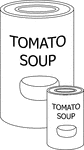2 Soup Can Cylinders

Illustration of 2 soup cans that are similar cylinders. The diameter and height of the smaller can is…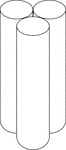3 Congruent Tangent Right Circular Cylinders

Illustration of 3 right congruent tangent circular cylinders. The height of all the cylinders is greater…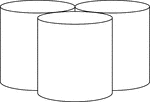3 Congruent Tangent Right Circular Cylinders

Illustration of 3 right congruent tangent circular cylinders.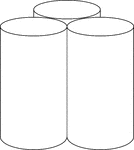3 Congruent Tangent Right Circular Cylinders

Illustration of 3 right congruent tangent circular cylinders. The height of all the cylinders is greater…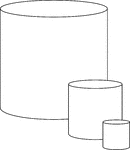3 Similar Cylinders

Illustration of 3 similar cylinders. The height and diameter in each successively smaller cylinder is…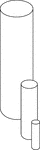3 Similar Cylinders

Illustration of 3 similar cylinders. The height and diameter in each successively smaller cylinder is…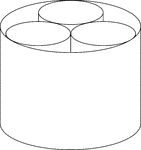3 Smaller Cylinders In A Larger Cylinder

A large cylinder containing 3 smaller congruent cylinders. The small cylinders are externally tangent…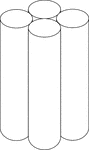4 Congruent Tangent Right Circular Cylinders

Illustration of 4 congruent tangent right circular cylinders. The height of all the cylinders is greater…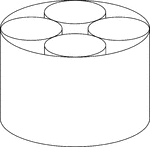4 Smaller Cylinders In A Larger Cylinder

A large cylinder containing 4 smaller congruent cylinders. The small cylinders are externally tangent…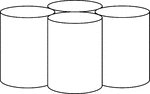4 Tangent Cylinders

Illustration of 4 congruent cylinders with diameters less than the height. The cylinders are externally…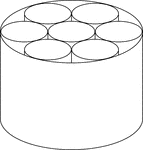7 Smaller Cylinders In A Larger Cylinder

A large cylinder containing 7 smaller congruent cylinders. The small cylinders are externally tangent…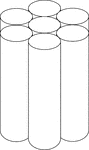7 Tangent Cylinders

Illustration of 7 congruent cylinders with diameters less than the height. 6 of the cylinders are equally…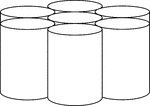7 Tangent Cylinders

Illustration of 7 congruent cylinders with diameters less than the height. 6 of the cylinders are equally…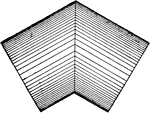Intersecting Cylinders

Illustration of the intersection of 2 cylinders of equal diameter.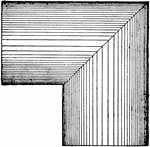Intersecting Cylinders

Illustration of the intersection of 2 cylinders of equal diameter.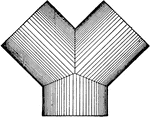Intersecting Cylinders

Illustration of the intersection of 3 cylinders of equal diameter.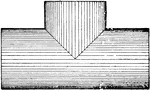Intersecting Cylinders

Illustration of the intersection of 2 cylinders of equal diameter.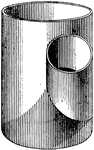Intersecting Cylinders

Illustration of the intersection of 2 cylinders of unequal diameter.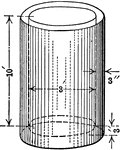Cylindrical Water Tank

Cylindrical water tank with a height of 10 ft., thickness of 3 inches, and diameter of 3 feet. The diagram…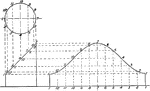Projections and Development of a Truncated Cylinder

Projections and development of a truncated cylinder.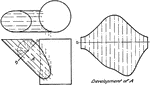Two Cylinders with Axes Not Intersecting

"If the cylinder A were to be developed a right section as at S—S would have to be taken, whose…